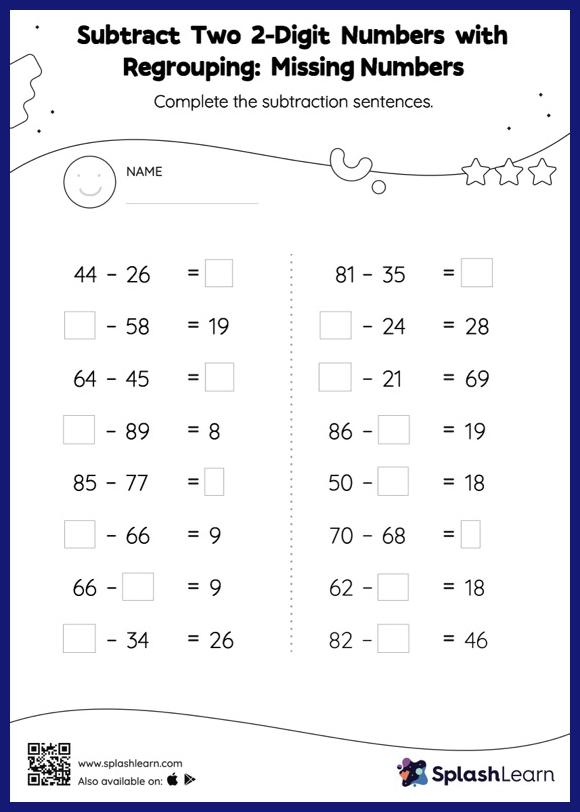# Subtract Two 2-Digit Numbers with Regrouping: Missing Numbers Worksheet

Home > Subtract Two 2-Digit Numbers with Regrouping: Missing NumbersChildren use their math skills to complete this subtract two 2-digit numbers with regrouping worksheet. Students use the count back strategy or the relationship between addition and subtraction to find the missing number in this worksheet. They also need to regroup numbers to get to the answer in subtract two 2-digit numbers with regrouping worksheet.